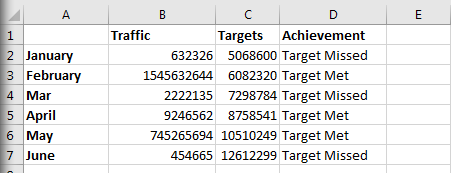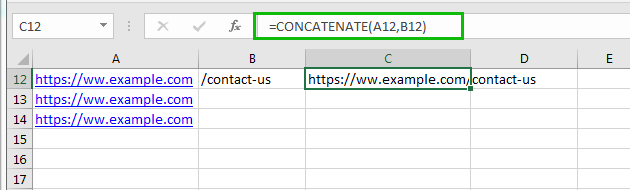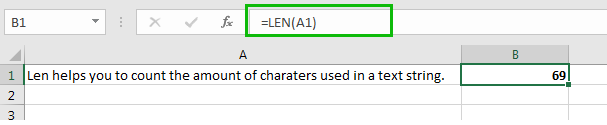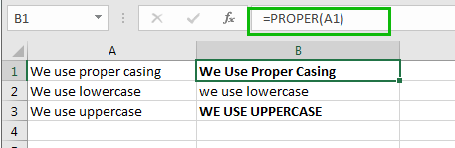# Excel Formulas Every Digital Marketer Should Know

16 August 2018 | 0 comments | Posted by Shamima Ahmed in nichemarket AdviceThe Digital Marketing life is hard as it is without having to worry about impressing the boss with complex and intricated formulas to show off your great progress.

Lucky we have Microsoft Excel and Google Sheets which, both nifty software tools to help us crunch numbers and then make some pretty cool visualisations.

## Why use formulas?

I'm a firm believer in making life easier by being a little bit smarter. Sometimes it may take a little more time to set up formulas in your sheet but once they implemented, you can literally use them for years to come and will save you a bunch of hours.

I can't express to you how much time I manage to save on daily tasks. I've literally user excel to check for duplication, find crazy parameters and even autogenerate XML sitemaps and on page tags like Meta and canonical tags.

There is literally no end to what you can do with this pool. Don't underestimate it's power, with the right formulas you can literally blow your own mind!!

## The most comprehensive excel cheat sheet

This blog will mainly focus on formulas to help you yield useful stats in your reports stats in your reports. If you are looking for some formatting solutions in MS Excel and Google Sheets take a look at this post.

### Basic built-in formulas for number crunching

If You already familiar with the basics, you can skip this part. More for newbs who need a bit of an intro to the power of Excel formulas.

#### Average:

This quickly Allows you to calculate the average of a set of numbers. Example formula is as follows: `=Average(A1:A7)` *Where A1:A7 is the range of cells chosen. Edit range to suit your target cells.

#### Sum:

This quickly Allows you to calculate the sum of a set of numbers. Example formula is as follows: `=Sum(A1:A7)` *Where A1:A7 is the range of cells chosen. Edit range to suit your target cells.

#### Max:

This quickly helps you to find the largest number in a range of numbers. Example formula is as follows: `=Average(A1:A100)` *Where A1:A100 is the range of cells chosen. Edit range to suit your target cells.

#### Min:

This quickly helps you to find the smallest number in a set of numbers. Example formula is as follows: `=Min(A1:F100)` *Where A1:F100 is the range of cells chosen. Edit range to suit your target cells.

#### Count:

This formula counts the number of cells that contain numbers in a specific range. Example formula is as follows: `=Count(B1:B17)` *Where B1:B17 is the range of cells chosen. Edit range to suit your target cells.

### Some More Useful Formulas for Number Crunching

These formulas are a little more advanced some are not standard and require a bit of thinking for calculation. Some are standard and built-in, and you may not think they would be useful, but trust me they are!!!

#### If statements:

This is a formula based on logic, it checks whether certain conditions are met, you can set teh outcome to show certain values if the statement is true or false. This handy if you looking to confirm almost anything in your data, such as:

• Check if targets were met
• Compare two sets of data to see if they match
• Find all users that have made a purchase in the last few months.

Of course, conditions can only be found if data is present in the sheet. If Statement Formula: `=if(logic_test,value_if_true,value_if_false)` If we look at the example below:D shows whether targets were met or not. We can quickly create the output in column D with the following formula: `=IF(B2>=C2,"Target Met","Target Missed")`Drag the formula down into the rest of column D and it will populate quickly.

#### Conversion Rate

Quickly Calculate How well your sales or lead generation tools ae performing, or any sort of action. Example formula is as follows: `=(number_of_target_interactions/total_interactions)*100` This can be used to calculate different types of outcoems, such as:

• Click through rates (clicks/total impressions)
• Sales (sales or transactions/total traffic)

#### % Growth

Growth rate can be used to caluculate to compare the present performance with past performance for almost any metric, such as: traffic, transactions, bookings, revenue, etc. `=((present-past)/past)*100`

### Useful Formulas for Manipulating Text

These formulas are a little more advanced some are not standard and require a bit of thinking for calculation. Some are standard and built-in, and you may not think they would be useful, but trust me they are!!!

#### Concatenate:

Concatenate is a super useful formula that allows you to join unlimited text strings from multiple cells. You can also add in manual bits of text. Example formula is as follows: `=Concatenate(text1,text2)`So if you wanted to combine some text from cell A12 and B12, you could do the following: ` =Concatenate(A12,A12)`

#### Len:

Len helps you to count the number of characters used in a text string. For example, if you wanted to count the number of characters in the sentence cell A1, you would use the following formula:

`=len(A1)`#### Proper, Lower and Upper

These function is very useful if you need to change the casing of sentences. Proper yields a capital letter for each word in the text string while lower and upper changes all the letters in the text string to lower and uppercase.

`=Proper(A1)`From the above, you can see Proper formula was applied for A1, while cells B2 and B3 contain formulas for lowercase and uppercase. They were implemented as follows.

`=Lower(A2) `

`=Upper(A3) `

If you have any tips to on how to implement formulas in a spreadsheet or would like us to demonstrate a specific method, comment below or contact us here.

Previous: {{ previousBlog.sTitle }}

Posted {{ previousBlog.dtDatePosting }}

Next: {{ nextBlog.sTitle }}

Posted {{ nextBlog.dtDatePosting }}

## You might also like### 83 Black Friday Software Deals 2023

21 November 2023

Posted by Che Kohler in Shopaholics

We put together a comprehensive list of software and digital business offering Black Friday discounts on their subscriptions or digital products this...### 5 Tips For Emergency Plumbing Situations

24 November 2023

Posted by Brigitte Evans in Constructive Criticism

As a home owner or tenant you need to prepared for plumbing issues and break downs or it can cause even more damage so make sure you follow these tip...

## Leave us a comment